Home --> Hintergrund: Inadequacy of the "Minimum Inhibitory Concentration" --> THE INADEQUACY OF MIC: ILLUSTRATIONS

# The Inadequacy of the Minimum Inhibitory Concentration

by Joachim Gruber

### Illustrations of Pharmacokinetic and Pharmacodynamic Models of the Antistaphylococcal Effects of Meropenem and Cloxacillin In Vitro and in Experimental Infection

##### AND ANNA E. A. DOUWES-IDEMA
Department of Infectious Diseases, University Hospital Leiden, 2300 RC Leiden, The Netherlands

ANTIMICROBIAL AGENTS AND CHEMOTHERAPY,
Oct. 1997, p. 2083?2088 Vol. 41, No. 10

### Abstract

Problem
When a bacteria population can be exposed to an antibiotic in-vitro in the same way as it is in vivo, the in-vitro experiments quantify the efficacy of the antibiotic.

When the antibiotic has strong side effects, one has to optimize between those effects and the risk of losing control over the bacteria population. In such critical cases an in-vitro model with only one parameter, the Minimum Inhibitory Concentration, may not be able to describe the development of an in-vitro bacteria population accurately enough. The Emax model presented here provides more accurate predictions, thus helping with the optimization. It can do so only at the expense of needing more information about the effect of the antibiotic, i.e. more model parameters. The pharmaceutic industry is willing to provide that information. It seems that the ball is now in the field of the treating physician: S/He needs to voice interest in those data.

Methods
The development of the number N[C, t] of the bacteria over time t under the influence of an antibiotic at concentration C is described by a non-linear first order differential equation. Four approximations of the growth or decay rate R[C, t] are used.

1. In the traditional MIC model, the rate R is constant in time and independent of C, except at two threshold concentrations C = MIC (Minimum Inhibitory Concentration) and C = MBC (Minimum Bactericidal Concentration, MBC > MIC). When C increases across these thresholds, the rate jumps:
1. when -starting from C = 0- C passes through MIC, the rate jumps from a positive value, R, to 0, and
2. when C passes through MBC the rate jumps from 0 to - R.
2. In three so-called Emax models the rate R may depend on the antibiotic concentration C and the time t over which the bacteria have been exposed to the antibiotic.
1. In the "adaptation" model, the rate R is a smoothed version of the MIC model jump at C = MIC, and thus also independent of t, i.e. R[C]. The time dependency in R describes the process of adaptation of the bacteria population to the antibiotic with a simple exponential law.
2. In the two other Emax models the time dependency of R[C, t] is represented by an asymmetrical bell-shaped curve with a rate maximum ERat time tmax.
1. In the complete version of this model, both the rate maximum ER and its time tmax depend on C: The functions ER[C] and tmax[C] are so-called "Hill expressions", which are basically smoothed step functions.
2. In a simple version, tmax does not change with C. Only the rate maximum ER is concentration dependent as given by a "Hill expression" ER[C].
Results
• When the antibiotic (meropenem) dose is chosen so that the bacteria population neither grows nor decays, i.e. when the meropenem concentration C is by definition near MIC, the MIC model gives a number of surviving bacteria typically deviating from the experimental values by less than plus or minus one order of magnitude.
• At concentrations C >> MIC, the MIC model gives too large bacteria populations (typically by two or more orders of magnitudes), thus being a conservative model.
• Independent from the meropenem concentration C the (compared to the MIC model more sophisticated) Emax model predicts bacteria population sizes that deviate from the data by typically a factor of three.
The results have been calculated with a program written in Mathematica (version 3.0). The program is a useful tool for conveying a feeling for the quantitiative influence of the system parameters. The program and my examples can be read with the free MathReader: The Mathematica Notebook Reader.

## Pharmacokinetics, Pharmacodynamics and the Influence of the Immune System

• Pharmacokinetics calculates the distribution of the pharmaceutical within the body, i.e. in a system of interconnected compartments.
• Pharmacodynamics calculates the development over time of the bacteria population under the effect of a pharmaceutical.
(A) The pharmacokinetic model is potentially misleading to the unexperienced pharmacologist when
1. the data and paramters describing a certain compartment have not been determined (as is often the case for intracellular locations) and
2. the fraction of the bacteria population in that compartment is non-negligible.
(B) Two similar statements apply to pharmacodynamic models. The model might not help in directing the therapy and control the illness when
1. the dynamics of a bacteria population in a certain compartment under the influence of the pharmaceutical is unknown,
2. the reaction of the immune system to the combined action of the pharmaceutical and the bacteria cannot be quantified.
Therefore, the pharmaceutical has to be -and often is- chosen such that neither (A) nor (B) is relevant, meaning that -given over a fixed period valid for all of us- the drug kills off or inhibits the growth a relevant fraction of the bacteria population, and the immune system does the rest to restore our health.

For short, I will call this "the one size fits all concept", meaning that all of us will be cured by the same therapy. When this therapy fails and we are sure that our diagnosis was correct, we talk of "niches" into which the bacteria can withdraw and be out of reach of the drug and immune system. Niches have been demonstrated

• in technical systems, e.g. in water supply systems where one possible niche is a biofilm, as well as
• in living systems, e.g. in our sinus-maxillary floor.
Such "niches" are the subject of research and therefore not usually known to our treating physicians. We -as patients- need to call his attention to the forefront of research - ideally by finding the relevant medical literature with the help of e.g. Medline.

Treatment failure manifests itself in a continuing shift of body parameters from normal to borderline or "positive" for a more or less wide range of illnesses. In many cases we do not know the mechanism that produces the shift, but -from experience- we know how to interpret it. Our interpretation might be called a "global understanding".

Of such global character is a large part of our practical knowledge of the immune response that we apply in therapies. Here is an example in the context of a case of neuroborreliosis [Gruber, 2002]:

1. Whereas the antibiotic itself brings about only exponential changes of the number of bacteria,
2. the immune system is shown to effect temporal oscillations of the number of the bacteria, some of which are "self-organized".
A conservative antibiotic treatment regime
• must consider self-organized immune system oscillations a sign for an ongoing active infection [Burrascano 2003, Gruber 2003]. and
• can have its end only after the cessation of these oscillations.
A more sophisticated treatment would be accompanied by mathematical model calculations. When sufficiently quantitative microscopic models of the immune response are missing, one might conceptually attempt to describe the development with time t of the number N of bacteria with the following global non-linear differential equation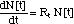(1)

where the non-linearity as incorporated in Rt summarizes the combined action of

1. the antibiotic and
2. the immune system
Such models have been presented in the literature and are being used to optimize the therapy. But they exceed the scope of this paper. So, the pharamcodynamics discussed here will ignore the influence of the immune system.

### I. The MIC Concept

By definition, a population of N bacteria exposed in vitro to a concentration C of an antibiotic stops growing when C is equal or higher than the Minimum Inhibitory Concentration (MIC).  When C drops below the MIC, the population grows with a rate R0, where R0 is now the fraction of the population that enters the cell division phase per unit time.

### I. 1 Non-Linear Differential Equation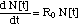(2)

### I. 2 Growth- and Kill-Rates R0

As soon as the antibiotic concentration exceeds the Minimum Bactericidal Concentration (MBC), the bacteria population decays.
In the case of a cell wall antibiotic, the straighforward model would assume that -when exposed to an antibiotic concentration C > MBC- any bacterium dies that tries to cell divide. Thus, the fraction of bacteria population that dies per unit time is equal to the fraction of that population that would cell divide in the absence of the cell wall antibiotic:
R[C > MIC] = - R[C < MIC] = - R0     (3)

With the definitions

R[C < MIC] = growth rate

- R[C > MIC] = kill rate,

eq. (3) can be written as

kill rate = growth rate

With this simplification, the equation describing the population dynamics in the presence or absence of an antibiotic can be written as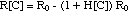(4)

where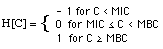(5)

### I. 3 Bacteria Population Dynamics N[C, t]

The bacteria population dynamics N[C, t] can be calculated from eq. (4) as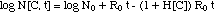("MIC model")(6)

It is shown in Figs. 1 and 1a.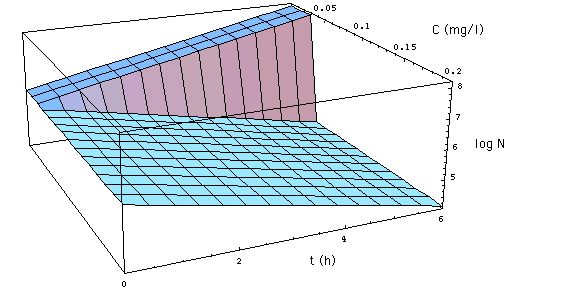Fig. 1: Development of a bacteria population as described by the MIC concept. x-axis is the time axis (t measured in hours)  y-axis gives the concentration C of meropenem in mg/l. The z-axis gives the logarithm of the number N[C, t] of S. aureus. N[C, t] as specified in (6), with R0 = 0.33 /h, MIC = 0.029 mg/l. The figure shows the development of the population when MBC = MIC.  (Click on Figure to see the development for MBC = 3 MIC. )
The population

• either grows with a growth rate R0 when the antibiotic concentration C < MIC,
• decays with the (kill) rate -R0 when C > MIC = MBC.

The publication of Mattie et al. quantifies the drawback of the MIC concept and proposes an improved pharmacodynamic model, which belongs to the class of so-called "Emax models".

### II. The Emax Model: Mathematical Formulation

The improved mathematical model presented by Mattie et al. is based on 3 assumptions:
1. The bacteria population dynamics is given by a non-linear differential equation.
2. The bacteria population reproduces -or dies- with a rate R[C, t], for which an empirical form is chosen. R[C, t] depends on 2 parameters ER[C] and tmax[C].
3. For the parameters ER[C] and tmax[C] empirical Hill expressions are chosen.

### II. 1 Non-Linear Differential Equation

The number of bacteria in the presence of the antibiotic is calculated with a non-linear first order differential equation similar to the one used for the MIC model: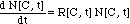(7)

### II. 2 Growth- and Kill-Rates R

For the rate R[C, t] the authors chose an empirical expression:(8)

where e is the base of the natural logarithm (e = 2.718). This empirical expression is visualized in Fig. 2.

A yet simpler approximation would replace the time dependence of R expressed in equation (8) with an adaptation term associated with the growth rate R0 [Mouton et al. 1997, Koop et al. 2000, Zhi et al. 1986].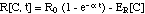(8m)

where 1/a is the time constant for the bacteria population adaptation to the antibiotic.

Note the slightly different definitions of ER[C] in eqs. (8) and (8m): ER[C] in eq. (8) has a factor e = 2.718 in front of it, whereas in eq. (8m) it does not.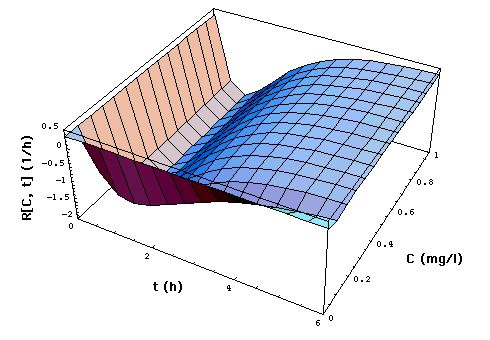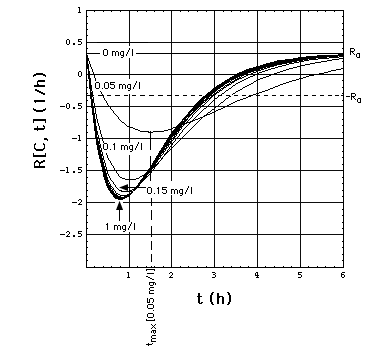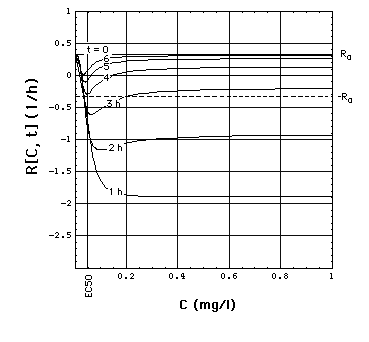Fig. 2: Staphylococcus aureus strain 1 rate R[C, t] as a function of time t and meropenem concentration C, calculated with "complete model" and the pharmacodynamic data eq. (16).
Clickon upper part of figure to see R[C, t] calculated with "complete", "adaptation" and MIC model.

Click here to see a "lake" with surface R[C, t] = 0 filled into the eq.(8) R[C, t] surface. The shoreline of this lake is the contour line R[C, t] = 0, and thus -by definition- the funtion MIC[t].

The lower part of the figure shows cross sections through the "complete" model surface in the upper part:

• left side: cross sections at constant C (C = 0, 0.05, 0.1, 0.15, 0.2, 0.25, ..., 1 mg/l) and
• right side: cross sections at constant t (t = 0, 1, 2, 3, 4, 5, 6h).
In the absence of meropenem (C = 0), S. aureus strain 1 growth rate is R0 = 0.33 /h. The minimum of R[C, t] as a function of t lies at tmax[C].

### II. 3 Bacteria Population Dynamics N[C, t]

The differential equation (7) is then solved by separation of the variables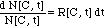(9)

and subsequent integration of both sides of the equation (9):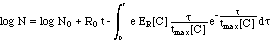(10)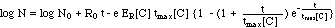("complete model")     (11)

A simpler versioin of eq. (11) would neglect the concentration dependence of tmax[C], replacing tmax[C] with the constant T.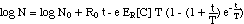("simplified model")     (12)

When the adaptation concept eq. (8m) is plugged into eq. (9) the integration yields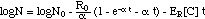("adaptation model")     (13)

### (4) Hill Expressions for ER[C] and tmax[C]

Hill expressions are being used to quantify the dependencies of ER and tmax on the antibiotic concentration C: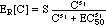(14) (click on equation to see graphical representation of ER[C] for meropenem)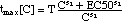(15) (click on equation to see graphical representation of tmax[C] for meropenem)

### III. Results

##### III. 1 Pharmacodynamic Data for Meropenem Emax Model
The following parameters are the ones that produced the best fits of the Emax models to the experimental data (Fig. 5)

 "complete" model" EC50 = 0.047 mg/l;     (16) S = 2.28 h-1; T = 0.79 h; s1 = 2.48; s2 = 1.6. "adaptation model"  EC50 = 0.047 mg/l;     (16m) S = 1.0 h-1; a = 0.5 h-1; s1 = 2.48;

##### III. 2 Population Dynamics in the Presence of Meropenem
Fig. 3 is a plot of the Emax population dynamics models ("complete" and "simplified") of S. aureus as a function of time t and meropenem concentration C.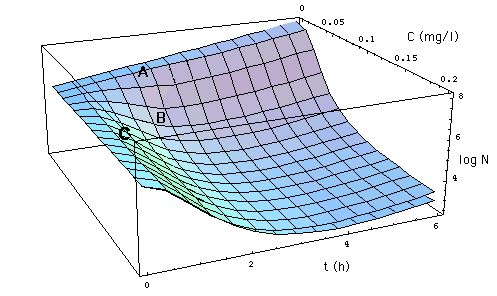Fig. 3: Development of number N[C, t] of S. aureus as a function of time t (x-axis) and meropenem concentration C (y-axis). As in Fig. 1, the z-axis gives the logarithm of the number N[C, t] of S. aureus. N[C, t] is specified

• in the "complete" model (11, lower surface) with empirical so-called Hill expressions (14) and (15) for ER[C] and tmax[C], respectively, and
• in the "simplified" model (12, lower surface), where an empirical Hill expression is used only for ER[C], whereas tmax[C] = T (a constant).
##### III. 3  "Concentration-" and "Time-Dependent" Antibiotics
The Emax models Fig. 3, eqs. (11, 12) show that the effect of an antibiotic can be dose- or time-dependent, depending on the concentration of the antibiotic.
• At low antibiotic concentrations (e.g. C = 0.05 mg/l, point A in Fig. 3),
• the effect of the antiibiotic is called "concenrtation-dependent", meaning that
• increasing the antibiotic C while keeping the exposure time t constant (e.g. t = 2 h) will reduce the number N more effectively than increasing the exposure time. Hence, here
• Example: moving from initial position A {t = 2 h, C = 0.05 mg/l} to final position B {t = 2 h, C = 0.1 mg/l} reduces the population of S. aureus by approximately a factor of 20. A similar reduction of N cannot be achieved by inreasing the exposure time t.
• At high antibiotic concentrations (e.g. at C = 0.15 mg/l, point C in Fiig. 3) the situation is reversed and the effect is called "time dependent":
• increasing concentration C effects only little change, whereas
• increasing t is much more effective.
Often, an antibiotic is characterized as having a concentration- or a time-dependent effect, when its usually applied dosage places it in the concentration-dependent region A or in the time-dependent region C, respectively [e.g. Goodman and Gilman's, Craig 1998]. This habit is misleading, since it is not the antibiotic that has that property but rather the dosage.

##### III. 4 Comparison of Population Dynamics Models
Fig. 4 compares the four population dynamics models
1. the "complete" model, eq. (11),
2. the "simplified" model, eq.(12),
3. the "adaptation" model, eq. (13). Note: S is re-fitted (S = 0.9 h) to improve position of the "adaptation model" surface N[C, t] relative to experimental data.
4. the "MIC model", eq. (4),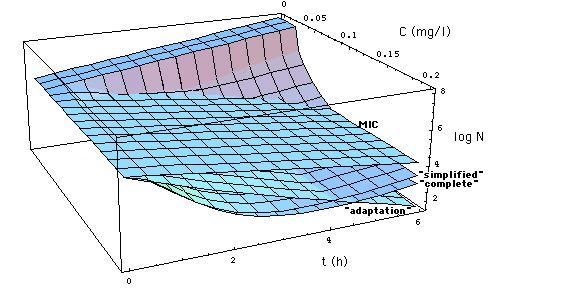Fig. 4: Comparison of MIC model (6, Fig. 1, MBC = MIC) with Emax models (11, 12, 13). Click on Figure to see comparison with MIC model in which MBC = 3 MIC.

The MIC model underestimates the effect of the antibiotic except at concentrations near EC50 = 0.047 mg/l.

Fig. 5 shows experimental data presented in the paper [Mattie et al.] and sections through Fig. 4 at constant meropenem concentrations C. The comparison with the "adaptation model" has been placed into a second layer Fig. 5a.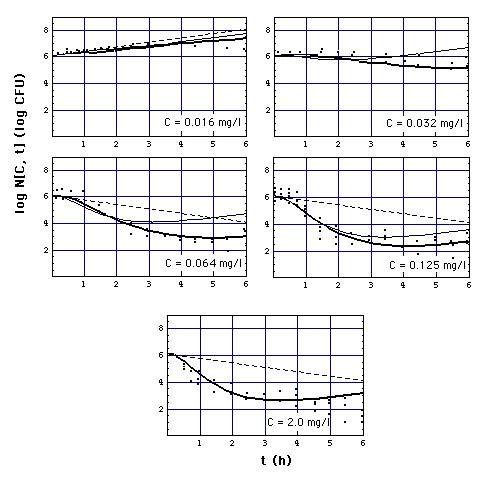Fig. 5: Number N[C, t] of S. aureus 1 organisms exposed to meropenem in vitro as a function of exposure time t, calculated with
• eq. (11) ("complete" Emax model, heavy curves),
• eq. (12) ("simplified" Emax model, normal curves), click on figure to see "adaptation" model eq. (13) instead of "simplified" model eq. (12).
• eq. (6) (MIC model with MIC = MBC = 0.03 mg/l, i.e. the  surface N[C,t] in Fig. 1, dashed curves).
• Points are experimentally determined numbers Nx[C,t] of S. aureus 1 (Fig.2 of [Mattie et al]),
Meropenem concentrations C are 0.016, 0.032, 0.064, 0.128, 2.0 mg/l. Model  (11) and (12) curves coincide at C = 2.0 mg/l. MIC = 0.029 mg/l is taken from Fig. 6, i.e. MIC is arbitrarily evaluated at t = 1 h. If bacteria would be killed only when they cell divide, their number would decrease as shown by lower dashed curve. Experimentally determined numbers (coinciding more or less with heavy curves) show a faster decay of population than that.

##### III. 5 Comparison Summary
1. The MIC model, eq. (6), has no explanation for the observed more rapid decay of the bacteria population at intermediate times:
• In the MIC model the cell wall antibiotic can at most kill all those cells that undergo cell division. So the rate of growth R0 and the maximum kill rate are conceptually bound to be identical.
• This is not how the "complete "model interprets the experimental data: In the fitting process the minimum of the R[C, t] curves at tmax (lower part of Fig. 2) is not fixed to lie within the range 0 < R[C, t] < - R0. The "complete" model represents the data best when (again see lower part of Fig. 2)
• the kill rate exceeds the growth rate R0 initially, i.e.

• R[C > EC50, t < tmax[C]] < - R0 (except for times near t = 0), and
• then declines to a value within that range at later times:

• 0 > R[C > EC50, t >> tmax[C]] > - R0.
The microbiological process responsible for the decline has not yet been fully understood. It is assumed that we see a phenotypical selection during the exposure to the antibiotic [Mouton et al.].
2. The simple Emax models, approximations (12) and (13), might be called "conservative" in the following sense: These models overestimate the number of surviving bacteria by 2 orders of magnitude (or less), as one can see in Figs. 4 and 5.
3. At large antibiotic concentrations (C >> EC50) as well as at large exposure times t >> tmax, the simplified Emax model eq. (12) differs only insignificantly from the complete Emax model eq. (11).
4. The larger the antibiotic concentrations, the less the complete Emax model and simplified Emax model curves deviate from each other.
5. At large exposure times t >> tmax and large antibiotic concentrations C >> EC50, the MIC model eq. (4) deviates from the full Emax model by less than an order of magnitude (Fig. 5).

### IV. REFERENCES

Burrascano JJ, "Diagnostic hints and treatment guidelines for Lyme and other tick borne diseases, 14. ed., 2002, COURSE DURING THERAPY
Craig WA, Pharmacokinetic/pharmacodynamic parameters: rationale for antibacterial dosing of mice and men. Clin. Infect. Dis., 1998, 26:1-10
Goodman and Gilman's "The Pharmacological Basis of Therapeutics", Chapter. 43, "Pharmacokinetic Factors", search inside book for "1160", from results select "on Page 1160".
Gruber J, Compartment models displaying Lyme disease symptom cycles, 2002.
Gruber J, Burrascano's Guidelines and Immune Response Modeling, 2003.
Koop AH, Neef C, van Gils SA. A mathematical model for the efficacy and toxicity of aminoglycoside (April 2003). Workshop "Predictive Value  of PK/PD models of antimicrobial drugs". Leiden University Medical Center, Leiden, The Netherlands, 4 - 5. September 2003.
Mouton JW, Vinks AA, Punt NC. Pharmacokinetic-Pharmacodynamic Modeling of activity of ceftazidime during continuous and intermittent infusion. Antimicrobial Agents and Chemotherapy 1997;1(4):733-738.
• model 3 in Mouton et al., page 734
• Vinks AA, Punt NC and Mouton JW. Pharmacokinetic-Pharmacodynamic Modeling of Bacterial Growth and Killing using the Modified Zhi Emax model. Workshop "Predictive Value  of PK/PD models of antimicrobial drugs". Leiden University Medical Center, Leiden, The Netherlands, 4 - 5. September 2003.
Zhi J, Nightingale CH, Quintiliani R. A pharmacodynamic model for the activity of antibiotics against microorganisms under nonsaturable conditions. J Pharm Sci 1986;75(11):1063-7.
Zhi JG, Nightingale CH, Quintiliani R. Microbial pharmacodynamics of piperacillin in neutropenic mice of systematic infection due to Pseudomonas aeruginosa. J Pharmacokinet Biopharm 1988;16(4):355-75.

### V. APPENDIX

##### V. 1 Dependence of MIC on Exposure Time
By definition, the Minimum Inhibitory Concentration is the antibiotic concentration C = MIC at which the bacteria population neither grows nor decreases, i.e. dN/dt = 0 in eq. (7). This is the case when R[C, t] = 0.

Solving the "complete" model rate expresssion eq. (8) for C, one can calculate the dependence of MIC on the time the bacteria population has been exposed to the antibiotic.

Eq. (17) or (17m) can be solved for MIC as a function of t. Mathematica can do this graphically. Fig. 6 is Mathematica's plot of the function MIC[t].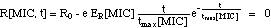(17)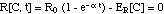(17m)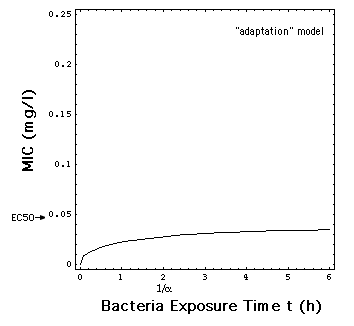Fig. 6: Dependence of MIC on the time t during which the bacteria population has been exposed in vitro to the antibiotic meropenem. The MIC's used in this paper are MIC[t = 1 h] = 0.029 mg/l, e.g the MIC in Fig.1 and MIC[t = 3 h] = 0.024 mg/l.

version August 8, 2012